﻿ 鄂尔多斯块体东北缘地区震源机制和应力场特征研究文章快速检索
 地震地磁观测与研究2021, Vol. 42Issue (1): 13-19  DOI: 10.3969/j.issn.1003-3246.2021.01.0030

### 引用本文BAO Jinzhe, WANG Shubo, HAO Meixian, et al. Research on focal mechanisms and stress field in the northeast edge of Ordos block[J]. Seismological and Geomagnetic Observation and Research, 2021, 42(1): 13-19. DOI: 10.3969/j.issn.1003-3246.2021.01.003.### 文章历史

Research on focal mechanisms and stress field in the northeast edge of Ordos block
BAO Jinzhe, WANG Shubo, HAO Meixian , HAN Xiaoming , ZHANG Hui , YIN Zhanjun , YANG Yanming
Earthquake Agency of Inner Mongolia Autonomous Regin, Hohhot 010010, China
Abstract: This paper selects 144 ML ≥ 2.5 earthquakes that occurred from January 2008 to April 2018 in the area of the northeast edge of ordos block (latitude 38.5°- 42.0°; longitude 109.5°-116.0°) and uses the method combining the amplitude ratio and P-wave initial motion analysis (FOCMEC) to obtain the focal mechanism solution for the area of northeast edge of Ordos block (the border area of Shanxi, Hebei, and Inner Mongolia). The stress field is obtained by using a damped stress inversion method (MSATSI) with a spatial resolution of 1°×1°. The results show that the main source types of earthquakes in the area of the northeast edge of Ordos block (the border area of Shanxi, Hebei, and Inner Mongolia) are normal faults and strike-slip type. The stress is mainly tension. There are shearing stress and tensile stress in some areas. The orientation of the maximum horizontal principal stress is mainly distributed in the NE direction.
Key words: focal mechanism    the border area of Shanxi, Hebei and Inner Mongolia    stress field    FOCMEC    MSATSI
0 引言

1 研究区域构造背景

2 震源机制解

 $\left\{ {\begin{array}{*{20}{l}} {{\mu _r} = \frac{1}{{4{\rm{ \mathsf{ π} }}\rho }} \cdot \frac{1}{{v_{\rm{p}}^3}} \cdot \frac{1}{r}\dot M\left({t - \frac{r}{{{v_{\rm{p}}}}}} \right){{\sin }^2}\theta \sin 2\phi }\\ {{\mu _\theta } = \frac{1}{{4{\rm{ \mathsf{ π} }}\rho }} \cdot \frac{1}{{v_{\rm{s}}^3}} \cdot \frac{1}{r}\dot M\left({t - \frac{r}{{{v_{\rm{S}}}}}} \right){{\sin }^2}\theta \cos \theta \sin 2\phi }\\ {{\mu _\phi } = \frac{1}{{4{\rm{ \mathsf{ π} }}\rho }} \cdot \frac{1}{{v_{\rm{s}}^3}} \cdot \frac{1}{r}\dot M\left({t - \frac{r}{{{v_{\rm{S}}}}}} \right){{\sin }^2}\theta \cos 2\theta } \end{array}} \right.$ (1)表 1 震源机制解类型 Table 1 Categories of tectonic stress regime for focal mechanism表 2 研究区内震源机制解分类 Table 2 Classification of focal mechanism solutions in the research area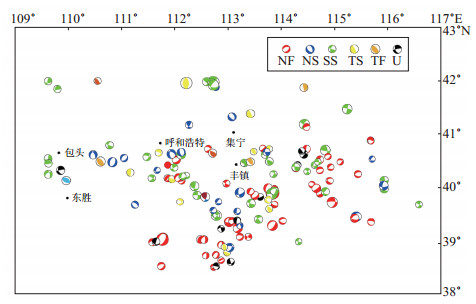图 2 震源机制解空间分布 Fig.2 Spatial distribution of focal mechanism solutions
3 应力场研究结果 3.1 阻尼系数的确定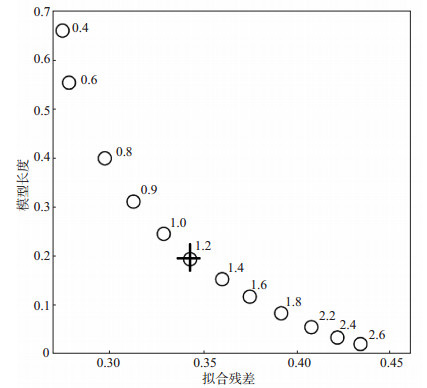图 3 模型长度—数据拟合误差 Fig.3 Model length-data fitting residual curve
3.2 鄂尔多斯块体东北缘区域应力场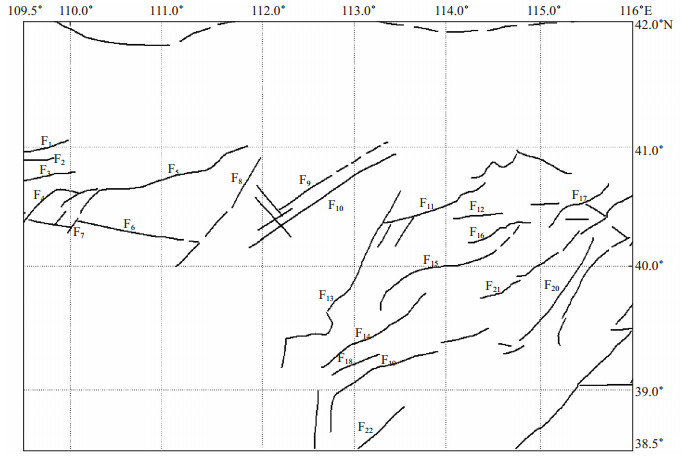图 1 研究区域地质构造 F1色尔腾山山前断裂；F2乌拉山北麓断裂；F3乌拉山山前断裂；F4包头断裂；F5大青山山前断裂；F6鄂尔多斯北缘断裂；F7达拉特旗断裂；F8和林格尔断裂；F9蛮汗山山前断裂；F10岱海—黄旗海盆地南缘断裂；F11天镇—阳高盆地北缘断裂；F12怀安镇盆地南断裂；F13口泉断裂；F14恒山北麓断裂；F15六棱山北麓断裂；F16阳原盆地北缘断裂；F17怀涿盆地北缘断裂；F18恒山南麓断裂；F19五台山北麓断裂；F20孙庄子—乌龙沟断裂；F21慰县盆地南缘断裂；F22系丹山山前断裂 Fig.1 Geological structure of the study region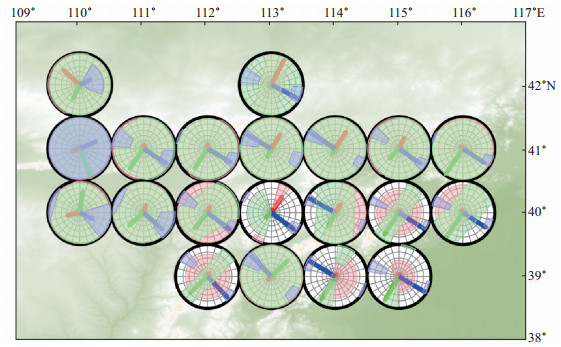图 4 应力场反演结果 圆圈反映各个反演格点的3个应力轴伍尔夫投影情况；红色线（P轴）表示最小主应力轴；绿色表示中间主应力轴；蓝色线（T轴）表示最大主应力轴 Fig.4 Stress field inversion results

 $R = \frac{{{\sigma _2} - {\sigma _1}}}{{{\sigma _3} - {\sigma _1}}}$ (2)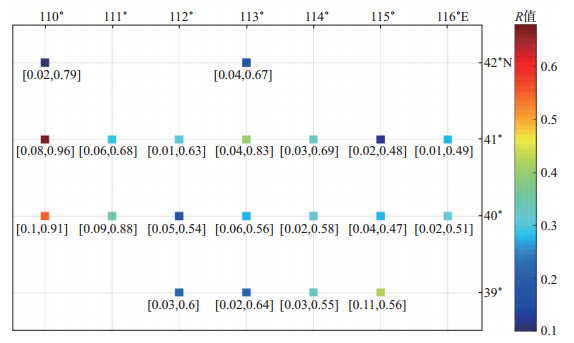图 5 相对应力R值空间分布 Fig.5 Spatial distribution of relative stress R-value
4 结论

 邓起东, 尤慧川. 鄂尔多斯周缘断陷盆地带的构造活动特征及其形成机制[M]//国家地震局地质研究所. 现代地壳运动研究(1). 北京: 地震出版社, 1985: 58-78. 邓起东, 程绍平, 闵伟, 等. 鄂尔多斯块体新生代构造活动和动力学的讨论[J]. 地质力学学报, 1999, 5(3): 13-21. DOI:10.3969/j.issn.1006-6616.1999.03.003 邓起东, 程绍平, 马冀, 等. 青藏高原地震活动特征及当前地震活动形势[J]. 地球物理学报, 2014, 57(7): 2025-2042. 范俊喜, 马瑾, 甘卫军. 鄂尔多斯地块运动的整体性与不同方向边界活动的交替性[J]. 中国科学(D辑), 2003, 33(Z1): 119-128. 高立新, 戴勇. 晋冀蒙交界地区地震活动性特征分析[J]. 华北地震科学, 2012, 30(3): 34-39. 国家地震局《鄂尔多斯周缘活动断裂系》课题组. 鄂尔多斯周缘活动断裂系[M]. 北京: 地震出版社, 1988. 郭祥云, 蒋长胜, 王晓山, 等. 鄂尔多斯块体周缘中小地震震源机制及应力场特征[J]. 大地测量与地球动力学, 2017, 37(7): 675-685. 李祥. 晋冀蒙交界地区一维速度模型及构造应力场研究[D]. 河北廊坊: 防灾科技学院, 2016. 梁尚鸿, 李幼铭, 束沛镒, 等. 利用区域地震台网P、S振幅比资料测定小震震源参数[J]. 地球物理学报, 1984, 27(3): 249-257. DOI:10.3321/j.issn:0001-5733.1984.03.005 盛书中. 鄂尔多斯块体周缘地壳应力场与断层面参数的研究[D]. 北京: 中国地震局地球物理研究所, 2015. 王化宇, 殷志刚, 金文臣. 晋冀蒙交界区6级地震前晋北区中小地震活动研究[J]. 地震地磁观测与研究, 2006, 27(6): 18-26. 武敏捷, 朱红彬, 林向东, 等. 由多地震活动参数分析晋冀蒙交界地区长期地震危险性[J]. 地震地磁观测与研究, 2014, 35(3/4): 57-62. Hardebeck J L, Michael A J. Damped regional-scale stress inversions: methodology and examples for southern California and the Coalinga aftershock sequence[J]. J Geophys Res, 2006, 111(B11): B11310. Kisslinger C, Bowman J R, Koch K. Procedures for computing focal mechanisms from local (SV/P)z data[J]. Bull Seismol Soc Am, 1981, 87(6): 1719-1730. Martínez-Garzón P, Kwiatek G, Ickrath M, et al. MSATSI: A MATLAB package for stress inversion combining solid classic methodology, a new simplified user-handling, and a visualization tool[J]. Seismol Res Lett, 2014, 85(4): 896-904. Snoke J A, Munsey J W, Teague A G, et al. A program for focal mechanism determination by combined use of polarity and SV-P amplitude ratio data[J]. Earthquake Notes, 1984, 55(3): 15-20.